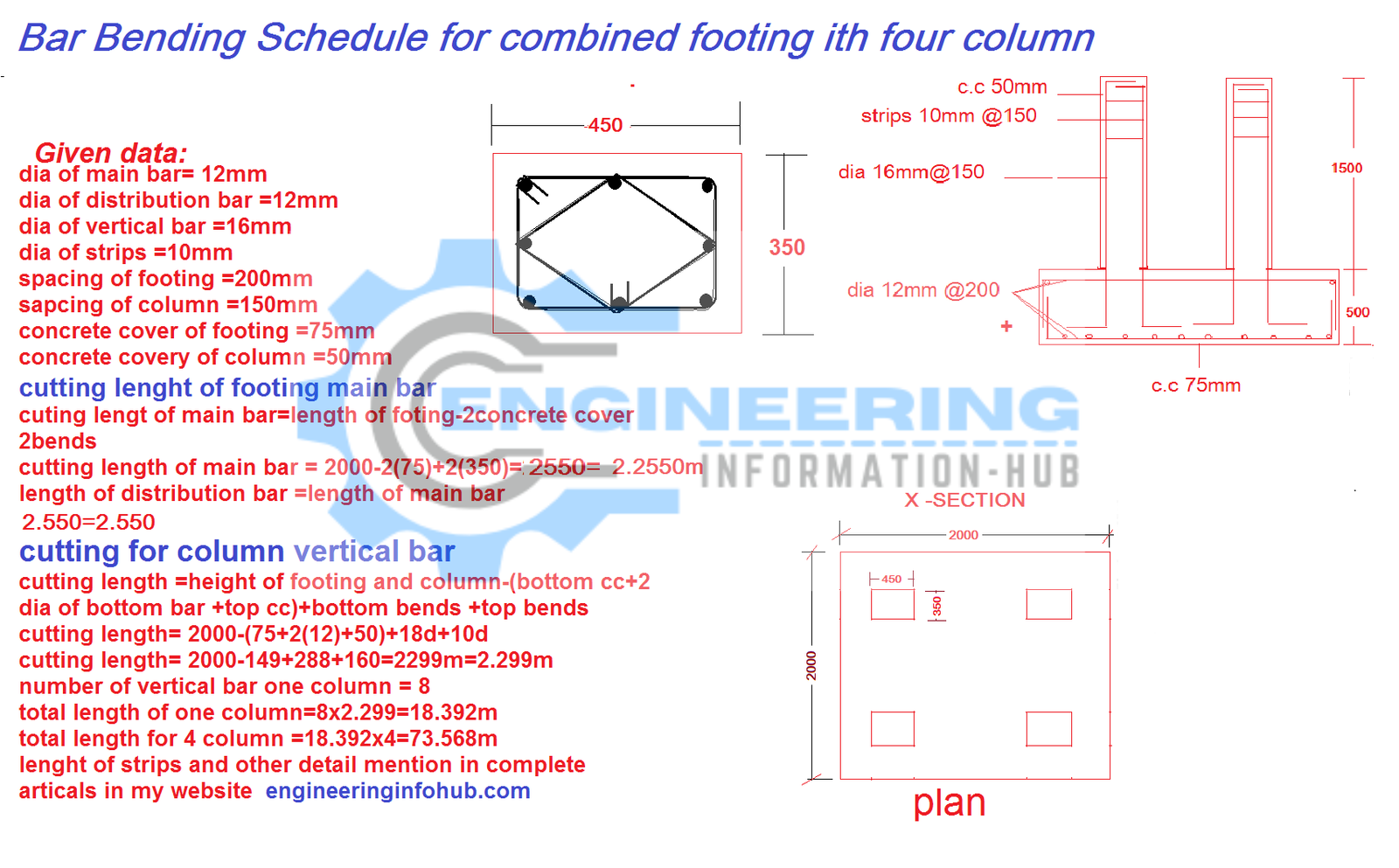Bar Bending Schedule

# Bar Bending Schedule For Combined Footing With Four Column

### What is the combined footing?

when we make the combined footing then the soil capacity is a low and small distance between two-column.

In this article, we will calculate the bar bending schedule for that footing has four-column and we will find the complete detail of the rebar step by step.

we have some given datadia of footing main bar =12mm

dia of footing distribution bar =12mm

spacing of footing=200mm

concrete cover of footing=75mm

dia of vertical bar in column=16mm

dia of strips =10mm

spacing of column=150mm

concrete cover of column=50mm

Now we calculate the cutting length of the main bar

# Bar Bending Schedule of Circular Column

cutting length of main bar=total length of footing-2c.c+2bends

cutting length of main bar=2000-2(75)+2(350)

cutting length of main bar=2000-150+700=2450=2.550m

Nos of main bar=total length/spacing+1

Nos of main bar=2000/200+1=11nos

total length of the main bar =length of one bar × nos of main bar

total length of the main bar =2.550×11=28.05m

weight of main bar= d²/162.25×total length

weight of main bar=12²/162.25×28.05=24.89kg=25kg

length of distribution bar=length of the main bar

28.05=28.05m

the total length of the main bar and distribution bat=56.10m

wight also same

25kg=25kg

total weight of 12mm bar for footing =50kg

the vertical bar of column

cutting length of vertical bar=total height of footing and column-bottom c.c -2dia of the bottom bar -top c.c +bottom bends+top bends

cutting length of vertical bar=2000-75-2(12)-50+288+160

cutting length of vertical bar=2000-149+448=2299=2.299m

nos of bar=8

total length of vertical bar =length of one bar ×nos of bar

the total length of vertical bar = 2.299×8=18.392m

weight of vertical bar=d²/162.25×total length

weight of vertical bar=16²/162.25×18.392=29.01kg=29kg

we have four column

Other post

# How to Calculate the Concrete of Volume for the Staircase

so

length of one column ×4=18.392×4=73.568m

weight of one column×4=29×4=56kg

Stips of column

nos of strips(Rectangle) =total height of column/spacing+1

nos of strips (rectangle)= 1500+150+1=11nos

cutting length of strips (Rectangle)=2side of 350+2side of 250+2bends (9d)

cutting length of strips (Rectangle)= 700+500+2(90)=1.380m

total length of strips=length of one stips×nos of strips

total length of strips=1.380×11=15.18m

weight of strips (Rectangle)=d²/162.25×total length

weight of strips (Rectangle)=10²/162.25×15.18=9.356kg=10kg

we have four column

so

length of one column ×4=60.72m

weight of one column×4=40kg

Now calculate the diagonal stips

length of strips =4sides of in diagonal +2bends(9d))

length of strips =4×215+2(9×10)=860+180=1040=1.040m

nos of strips =total height/spacing+1=1500/150+1=11nos

total length of strips=length of one strips ×nos of strips

total length of strips=1.040×11=11.44m

weight of stips =d²/162.25×total length=10²/162.25×11.44=7.05kg= 7kg

we have four column

length of one column stips×4=11.44×4=45.76m

weight of one column strips ×4=7×4=28kg

Now

total length of 12mm bar =56.10m

total weight of 12mm bar =50kg

total length of 16mm bar=73.56m

total weight of 16mm bar =56kg

total length of 10mm bar =68kg

total weight of 10mm bar =110kg

### Raja Numan

Hi, My name is Engr. Raja Numan author of Engineering Information Hub and I am a Civil Engineer by Profession and I've specialized in the field of Quantity Surveying, Land Surveying as QC Engineer in national and multinational companies of Pakistan & Saudi Arabia.

### Related Articles

1.Mubashar Rehan says:
2.Tanvir Raihan says: